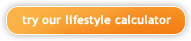# individuals - how it works## how we calculate emissions

The carbon dioxide footprint for individuals is based on the two areas of energy use that make up the majority of carbon dioxide emissions. The following calculations are based on data available at the website of the GHG protocol initiative.

## transportation

Automobile:
Inputs are for number of kilometers or miles driven and fuel efficiency of the car driven. Total number of kilometers driven per month is divided by miles driven per month is divided by miles per gallon to determine total number of gallons of fuel that are used each month.

If "small car" is chosen, fuel efficiency is estimated at 29 miles per gallon. For "medium size car," 26 miles per gallon is used, and for "large car, truck, or SUV" 21.5 miles per gallon is used. This information comes from the U.S. Environmental Protection Agency.

Airplane:
The number of miles traveled is multiplied by 1.61 to get kilometers traveled, which is then multiplied by .18 kgs CO2 per km to get total kgs CO2. 2.2046 to covert to pounds of CO2 multiply this number. We use the emissions factor (.18) for short haul flights used by the GHG Protocol Initiative.

## home energy

Electricity:
The number of kilowatt-hours is multiplied by the pounds of carbon dioxide produced per kilowatt-hour to get total pounds of CO2. The US average is 1.34 lbs of carbon dioxide per kilowatt-hour. Emissions factors used are from the US Department of Energy: Updated State Level Greenhouse Gas Emission Coefficients for Electricity Generation 1998-2000. These are currently being updated to the latest statistics available from DOE, dated April 2002.

Green power subscriber:
If green power sources that do not result in carbon emissions are used, the calculator reduces the carbon output due to electricity by the percentage of green power used. For example, if a green power supplier supplies 25% of the user’s electricity use, the carbon emission due to electricity is reduced by 25%.

Natural gas:
The units' input is converted to BTUs (British Thermal Units) from either therms (equal to 100,000 BTU) or thousand cubic feet (equal to 1,050,000 BTU). The resulting BTUs are multiplied by 0.0001304 lbs of carbon dioxide per BTU to get total pounds of CO2. The emissions factor is from US Department of Energy.

Heating oil:
Quantity is converted to gallons (1 liter = 0.2642 gallons) if it was in liters. Number of gallons is multiplied by 11.81 to get total kgs of CO2, which is multiplied by 2.205 to get pounds of CO2. The emissions factor is from US Department of Energy.

Propane:
Quantity is converted to gallons (1 liter = 0.2642 gallons) if it was in liters. Number of gallons is multiplied by 5.75 to get total kgs of CO2, which is multiplied by 2.205 to get pounds of CO2. The emissions factor is from US Department of Energy.

## results

 Pounds of CO2 emissions per month Below 507 lbs/mo Much less than average Between 507 and 1,013 lbs/mo Less than average Between 1,014 and 2,028 lbs/mo Average Between 2,029 and 2,535 lbs/mo More than average Above 2,535 lbs/mo Much more than average

The carbon dioxide footprint is the sum of all the above calculations, resulting in an estimate of the carbon dioxide that is produced by home energy use and transportation. The result is compared against an estimated monthly per capita US average of 1,521 pounds resulting from transportation and home energy emissions. This amount represents roughly 40% of per capita emissions in the U.S.

For comparison purposes, any result within 33% of the 1,521 pounds per month is considered to be an "average" amount. Between 33% and 67% above or below the 1,521 pounds per month is considered "more than average" or "less than average," respectively. A result greater than 66% above or below the 1,521 pounds per month is considered "much more than average" or "much less than average," respectively.